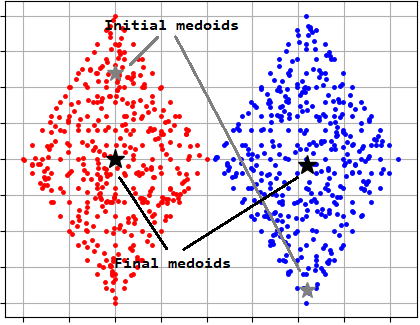pyclustering  0.10.1 pyclustring is a Python, C++ data mining library.
pyclustering.cluster.kmedoids.kmedoids Class Reference

Class represents clustering algorithm K-Medoids (PAM algorithm). More...

## Public Member Functions

def __init__ (self, data, initial_index_medoids, tolerance=0.0001, ccore=True, **kwargs)
Constructor of clustering algorithm K-Medoids. More...

def process (self)
Performs cluster analysis in line with rules of K-Medoids algorithm. More...

def predict (self, points)
Calculates the closest cluster to each point. More...

def get_clusters (self)
Returns list of allocated clusters, each cluster contains indexes of objects in list of data. More...

def get_medoids (self)
Returns list of medoids of allocated clusters represented by indexes from the input data. More...

def get_cluster_encoding (self)
Returns clustering result representation type that indicate how clusters are encoded. More...

## Detailed Description

Class represents clustering algorithm K-Medoids (PAM algorithm).

PAM is a partitioning clustering algorithm that uses the medoids instead of centers like in case of K-Means algorithm. Medoid is an object with the smallest dissimilarity to all others in the cluster. PAM algorithm complexity is $$O\left ( k\left ( n-k \right )^{2} \right )$$.

There is an example where PAM algorithm is used to cluster 'TwoDiamonds' data:

from pyclustering.cluster.kmedoids import kmedoids
from pyclustering.cluster.center_initializer import kmeans_plusplus_initializer
from pyclustering.cluster import cluster_visualizer
from pyclustering.samples.definitions import FCPS_SAMPLES
# Load list of points for cluster analysis.
# Initialize initial medoids using K-Means++ algorithm
initial_medoids = kmeans_plusplus_initializer(sample, 2).initialize(return_index=True)
# Create instance of K-Medoids (PAM) algorithm.
kmedoids_instance = kmedoids(sample, initial_medoids)
# Run cluster analysis and obtain results.
kmedoids_instance.process()
clusters = kmedoids_instance.get_clusters()
medoids = kmedoids_instance.get_medoids()
# Print allocated clusters.
print("Clusters:", clusters)
# Display clustering results.
visualizer = cluster_visualizer()
visualizer.append_clusters(clusters, sample)
visualizer.append_cluster(initial_medoids, sample, markersize=12, marker='*', color='gray')
visualizer.append_cluster(medoids, sample, markersize=14, marker='*', color='black')
visualizer.show()Fig. 1. K-Medoids (PAM) clustering results 'TwoDiamonds'.

Metric for calculation distance between points can be specified by parameter additional 'metric':

# create Minkowski distance metric with degree equals to '2'
metric = distance_metric(type_metric.MINKOWSKI, degree=2)
# create K-Medoids algorithm with specific distance metric
kmedoids_instance = kmedoids(sample, initial_medoids, metric=metric)
# run cluster analysis and obtain results
kmedoids_instance.process()
clusters = kmedoids_instance.get_clusters()

Distance matrix can be used instead of sequence of points to increase performance and for that purpose parameter 'data_type' should be used:

# calculate distance matrix for sample
matrix = calculate_distance_matrix(sample)
# create K-Medoids algorithm for processing distance matrix instead of points
kmedoids_instance = kmedoids(matrix, initial_medoids, data_type='distance_matrix')
# run cluster analysis and obtain results
kmedoids_instance.process()
clusters = kmedoids_instance.get_clusters()
medoids = kmedoids_instance.get_medoids()

Definition at line 26 of file kmedoids.py.

## ◆ __init__()

 def pyclustering.cluster.kmedoids.kmedoids.__init__ ( self, data, initial_index_medoids, tolerance = 0.0001, ccore = True, ** kwargs )

Constructor of clustering algorithm K-Medoids.

Parameters
 [in] data (list): Input data that is presented as list of points (objects), each point should be represented by list or tuple. [in] initial_index_medoids (list): Indexes of intial medoids (indexes of points in input data). [in] tolerance (double): Stop condition: if maximum value of distance change of medoids of clusters is less than tolerance than algorithm will stop processing. [in] ccore (bool): If specified than CCORE library (C++ pyclustering library) is used for clustering instead of Python code. [in] **kwargs Arbitrary keyword arguments (available arguments: 'metric', 'data_type', 'itermax').

Keyword Args:

• metric (distance_metric): Metric that is used for distance calculation between two points.
• data_type (string): Data type of input sample 'data' that is processed by the algorithm ('points', 'distance_matrix').
• itermax (uint): Maximum number of iteration for cluster analysis.

Definition at line 100 of file kmedoids.py.

## ◆ get_cluster_encoding()

 def pyclustering.cluster.kmedoids.kmedoids.get_cluster_encoding ( self )

Returns clustering result representation type that indicate how clusters are encoded.

Returns
(type_encoding) Clustering result representation.
get_clusters()

Definition at line 248 of file kmedoids.py.

## ◆ get_clusters()

 def pyclustering.cluster.kmedoids.kmedoids.get_clusters ( self )

Returns list of allocated clusters, each cluster contains indexes of objects in list of data.

process()
get_medoids()

Definition at line 224 of file kmedoids.py.

## ◆ get_medoids()

 def pyclustering.cluster.kmedoids.kmedoids.get_medoids ( self )

Returns list of medoids of allocated clusters represented by indexes from the input data.

process()
get_clusters()

Definition at line 236 of file kmedoids.py.

## ◆ predict()

 def pyclustering.cluster.kmedoids.kmedoids.predict ( self, points )

Calculates the closest cluster to each point.

Parameters
 [in] points (array_like): Points for which closest clusters are calculated.
Returns
(list) List of closest clusters for each point. Each cluster is denoted by index. Return empty collection if 'process()' method was not called.

An example how to calculate (or predict) the closest cluster to specified points.

from pyclustering.cluster.kmedoids import kmedoids
from pyclustering.samples.definitions import SIMPLE_SAMPLES
# Load list of points for cluster analysis.
# Initial medoids for sample 'Simple3'.
initial_medoids = [4, 12, 25, 37]
# Create instance of K-Medoids algorithm with prepared centers.
kmedoids_instance = kmedoids(sample, initial_medoids)
# Run cluster analysis.
kmedoids_instance.process()
# Calculate the closest cluster to following two points.
points = [[0.35, 0.5], [2.5, 2.0]]
closest_clusters = kmedoids_instance.predict(points)
print(closest_clusters)

Definition at line 178 of file kmedoids.py.

## ◆ process()

 def pyclustering.cluster.kmedoids.kmedoids.process ( self )

Performs cluster analysis in line with rules of K-Medoids algorithm.

Returns
(kmedoids) Returns itself (K-Medoids instance).
Remarks
Results of clustering can be obtained using corresponding get methods.
get_clusters()
get_medoids()

Definition at line 137 of file kmedoids.py.

The documentation for this class was generated from the following file:
pyclustering.cluster.kmedoids
Cluster analysis algorithm: K-Medoids.
Definition: kmedoids.py:1
pyclustering.cluster.center_initializer
Collection of center initializers for algorithm that uses initial centers, for example,...
Definition: center_initializer.py:1
pyclustering.cluster
pyclustering module for cluster analysis.
Definition: __init__.py:1
pyclustering.utils.calculate_distance_matrix
def calculate_distance_matrix(sample, metric=distance_metric(type_metric.EUCLIDEAN))
Calculates distance matrix for data sample (sequence of points) using specified metric (by default Eu...
Definition: __init__.py:54
pyclustering.utils.metric.distance_metric
Distance metric performs distance calculation between two points in line with encapsulated function,...
Definition: metric.py:52
pyclustering.utils
Utils that are used by modules of pyclustering.
Definition: __init__.py:1